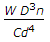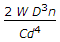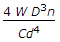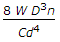# Mechanical Engineering - Strength of Materials

### Exercise :: Strength of Materials - Section 7

26.

When a rectangular beam is loaded transversely, the maximum tensile stress is developed on the

 A. top layer B. bottom layer C. neutral axis D. every cross-section

Explanation:

No answer description available for this question. Let us discuss.

27.

The compressive strength of brittle materials is __________ its tensile strength.

 A. equal to B. less than C. greater than

Explanation:

No answer description available for this question. Let us discuss.

28.

When a closely-coiied helical spring of mean diameter (D) is subjected to an axial load (W), the deflection of the spring (δ) is given by (where d = Diameter of spring wire, n = No. of turns of the spring, and C = Modulus of rigidity for the spring material)

 A.B.C.D.Explanation:

No answer description available for this question. Let us discuss.

29.

In spring balances, the spring is used

 A. to apply forces B. to measure forces C. to absorb shocks D. to store strain energy

Explanation:

No answer description available for this question. Let us discuss.

30.

According to Euler's column theory, the crippling load of a column is given by p = π2 EI/Cl2. In the Euler's formula, the value of C for a column with both ends fixed is 4.

 A. Agree B. Disagree